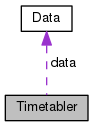Timetabler
Timetabler Class Reference

Class for time tabler. More...

`#include <time_tabler.h>`

Collaboration diagram for Timetabler:[legend]

## Public Member Functions

Timetabler ()
Constructs the Timetabler object.

~Timetabler ()
Destroys the object, and deletes the solver.

void addClauses (const std::vector< CClause > &, int)
Adds clauses to the solver with specified weights. More...

void addClauses (const Clauses &, int)
Adds clauses to the solver with specified weights. More...

bool checkAllTrue (const std::vector< Var > &)
Checks if a given set of variables are true in the model returned by the solver. More...

bool isVarTrue (const Var &)
Determines if a given variable is true in the model returned by the solver. More...

SolverStatus solve ()
Calls the solver to solve for the constraints. More...

Var newVar ()
Calls the formula to issue a new variable and returns it. More...

Lit newLiteral (bool sign=false)
Calls the formula to issue a new literal and returns it. More...

void printResult (SolverStatus)
Prints the result of the problem.

void displayTimeTable ()
Displays the generated time table.

void displayUnsatisfiedOutputReasons ()
Displays the reasons due to which the formula could not be satisfied, if applicable.

Adds unit soft clauses for the high level variables to the solver.

Adds high level clauses for predefined constraints. More...

Adds high level custom constraint clauses. More...

void writeOutput (std::string)
Writes the generated time table to a CSV file. More...

Adds unit clauses corresponding to existing assignments given in the input to the solver.

void addToFormula (vec< Lit > &, int)
Add a given vec of literals with the given weight to the formula. More...

Add single literal to the formula. More...

void displayChangesInGivenAssignment ()
Displays the changes that have been made to the default assignment given by the user as input by the solver.

Data data

## Detailed Description

Class for time tabler.

This class is responsible for handling the solver. This accepts constraints, adds it to the MaxSATFormula, calls the solver, and interprets and displays the result. It is also responsible for writing the output to a CSV, and creating new literals or variables in the solver when requested and returning them.

## Member Function Documentation

 void Timetabler::addClauses ( const std::vector< CClause > & clauses, int weight )

Adds clauses to the solver with specified weights.

A negative weight implies that the clauses are hard, and a zero weight implies that the clauses are not added to the solver.

Parameters
 [in] clauses The clauses [in] weight The weight
 void Timetabler::addClauses ( const Clauses & clauses, int weight )

Adds clauses to the solver with specified weights.

A negative weight implies that the clauses are had, and a zero weight implies that the clauses are not added to the solver.

Parameters
 [in] clauses The clauses [in] weight The weight
 void Timetabler::addHighLevelConstraintClauses ( PredefinedClauses clauseType )

Adds high level clauses for predefined constraints.

For each predefined constraint C, a variable x is created, and a hard clause x->C is added to the solver. A unit clause with x is added as a soft clause with weight as specified for the constraint.

Parameters
 [in] clauseType The clause type
 void Timetabler::addHighLevelCustomConstraintClauses ( int index, int weight )

Adds high level custom constraint clauses.

For each custom constraint C, a variable x is created, and a hard clause x->C is added to the solver. A unit clause with x is added as a soft clause with weight as specified for the constraint.

Parameters
 [in] index The index of the custom constraint variable [in] weight The weight of the custom constraint
 void Timetabler::addToFormula ( vec< Lit > & input, int weight )

Add a given vec of literals with the given weight to the formula.

A negative weight implies that the clauses are had, and a zero weight implies that the clauses are not added to the solver.

Parameters
 input The input [in] weight The weight
 void Timetabler::addToFormula ( Lit input, int weight )

Add single literal to the formula.

Parameters
 [in] input The input [in] weight The weight
 bool Timetabler::checkAllTrue ( const std::vector< Var > & inputs )

Checks if a given set of variables are true in the model returned by the solver.

Parameters
 [in] inputs The input variables
Returns
True, if all variables are True, False otherwise
 bool Timetabler::isVarTrue ( const Var & v )

Determines if a given variable is true in the model returned by the solver.

Parameters
 [in] v The variable to be checked
Returns
True if variable true, False otherwise
 Lit Timetabler::newLiteral ( bool sign = `false` )

Calls the formula to issue a new literal and returns it.

Parameters
 [in] sign The sign, whether the literal contains a sign with the variable
Returns
The Lit corresponding to the new Var added to the formula
 Var Timetabler::newVar ( )

Calls the formula to issue a new variable and returns it.

Returns
The new Var added to the formula
 SolverStatus Timetabler::solve ( )

Calls the solver to solve for the constraints.

Returns
True, if all high level variables were satisfied, False otherwise
 void Timetabler::writeOutput ( std::string fileName )

Writes the generated time table to a CSV file.

Parameters
 [in] fileName The file path of the output CSV file

## Member Data Documentation

 Data Timetabler::data

Stores all the field and input data obtained by the parser

The documentation for this class was generated from the following files: